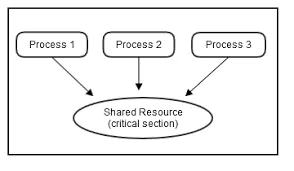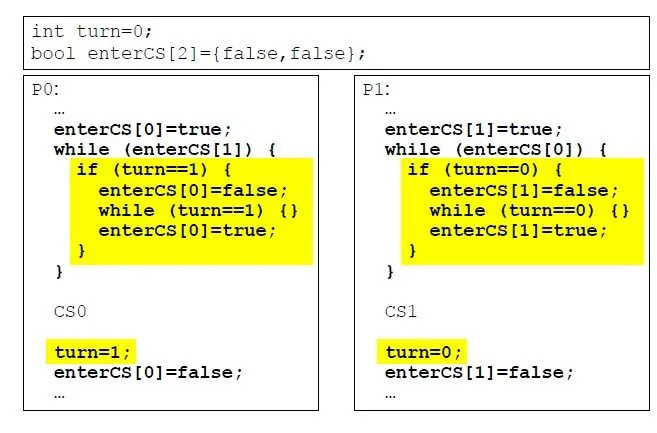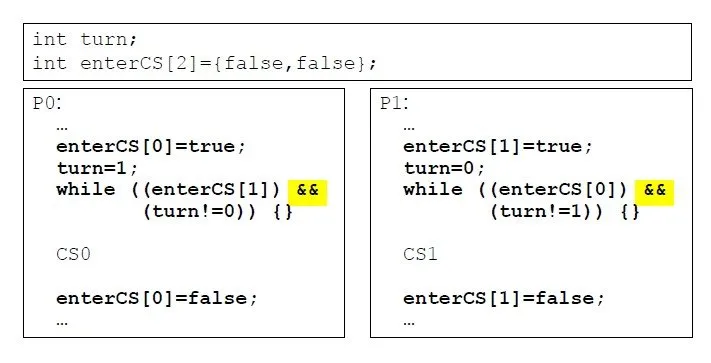# Networking - C Process/Thread Synchronization in LinuxHello it's me again! Today we will get into Synchronization of Processes or Threads in C Linux. I will start off explaining why we need Synchronization talking about some Theory stuff and then we will get into the implementation part. I will start off with some algorithms that work for 2 Processes/Threads and then get into Semaphores that are much better to use.

# Why Synchronization?

When we talked about Processes and Threads we saw in some examples that the output could become different if more then 1 processes/threads run at the same time, because of the process/thread switch inside of the CPU. But, there the order didn't gave any errors.

### Example:

To make it more clearly let's get into a small example. Suppose we want to calculate the sum of 1 + 2 + .... + 100 using 2 Processes/Threads that calculate one the odd and the other the even numbers sum. So, the first Process/Thread will calculate the sum 1 + 3 + ... + 99 and the second one the sum of  2 + 4 + ... + 100. But, we don't want to store the result temporary in each Process/Thread, but directly on a shared variable. If we don't use synchronization to prevent the 2 Processes/Threads access the shared variable together we will end up having the wrong answer.

### Assembly Explanation:

In C this simple assignment looks like one line of Code, but to understand this better we need to get into Assembly Code! When adding a value to an variable we do the following:

• Load the variable into a register (lw)
• Store the variable again, storing the value of the register (sw)

This 3 Instructions can be blocked at any time and nothing ensures us that they will all be called before a process/thread switch! There are also some functions that the operation system makes sure to finish before switching, but a basic addition can be blocked in any of the 3 steps. So, as you can see if the variables value gets loaded and we also have added to it and then a switch happens and the same register changes value during this switch, we will end up with a wrong storing value!

### Critical Section:

This addition we talked about in the example is a so called Critical Section! Any part of our Code that we have to ensure finishing before switching is a Critical Section. In this Section we mostly have something shared between Processes/Threads like a shared Variable, Memory etc. that more then 1 Processes/Threads have access to read to/write from

A Critical Section needs to:

• Let a Process/Thread enter the Section if it desires to after some waiting (progress) without bringing a deadlock and without starvation.

To do this we will have a entry code that prevents the entry of any other Process/Thread into the Critical Section if another Process/Thread is already in there. And we will also have some exit code that frees one of the blocked Processes/Threads.

So, our Structure looks like this:

``// mutual exlusion with entry code``
``// critical section``
``// progress with exit code``

# Synchronization Algorithms for 2 Processes:

2 pretty good Algorithms that work for 2 processes/threads are the Dekker and Peterson Algorithms. I will not get in depth so much, but simply give you a pseudocode for both and also explain why they work right!

### Dekker:The Dekker algorithm is using a integer turn that defines the process that has the turn currently and a boolean array that specifies the desire of entry into the Critical Section. This algorithm prevents the other process to enter checking the boolean value of the other process. Progress is being made setting the turn value to the other process and also setting the boolean value to false making the other process stop busy waiting and then get into the Critical Section. We also prevent starvation having the turn value be set to the other process.

### Peterson:Peterson uses the same variables, but this time gives the other process/thread the change to get inside if it desires to. So, we prevent insertion checking if the other process has the desire and also if the turn is not our turn. We then assure progress by making the other process stop busy waiting setting our bool to false. We also prevent starvation, cause even if the other process resets its boolean to true after false, it will also sets the turn to the other process and so unblock the other first, making the other get into the CS!

The problem with those algorithms is that a lot of CPU time gets wasted in busy waiting, because of the endless check of a specific condition inside of a while loop!

# Semaphores:

A semaphore is a synchronization tool. It has a value that can be increased (up/signal) or decreased (down/wait). The functions up/signal and down/wait are being run by the operation system and prevent switching to occur in between. The main library used in C Linux is called semaphore.h and it contains everything we need to synchronize processes. The library pthread.h that we talked about in Threads also contains it's own semaphores and I will talk about both together!

The main functions needed are:

• init(sem s, int val) -> that initializes a semaphore with the value val
• down(sem s) -> that decreases the value of the semaphore and blocks the process if we have a value of 0 or less
• up(sem s) -> that increases the value of the semaphore unblocking one of the processes waiting

We have 2 basic types of semaphores:

• General usage with a integer value that are used for counting
• Binary or Mutexes with a value of 0 or 1 that are used for mutual exclusion

In Implementation with semaphore.h we use the following:

``sem_t s; // define semaphore variable``
``sem_init(&s, 0, val); // initialize semaphore to val (0 or 1 for mutex)``
``sem_wait(&s); // down semaphore``
``sem_post(&s); // up semaphore``
``sem_destroy(&s); // destroy semaphore``

In Implementation with pthread.h mutexes we do:

``pthread_mutex_t mutex; // define mutex variable``
``pthread_mutex_init(&mutex, NULL); // initialize mutex (value 1)``
``pthread_mutex_lock(&mutex); // wait/down mutex``
``pthread_mutex_unlock(&mutex); // signal/up mutex``
``pthread_mutex_destroy(&mutex); // destroy mutex``

# Example Implementation:

For you to understand it a little bit let's synchronize the Multi-Thread Code from last time in Threads! I will change the printing with an increment and print of a shared variable and make the Threads count to 100! The Critical Section will be the incrementation of this shared variable that we will put inside of a lock and unlock mutex block. I will also make sure that all threads have been created before starting counting making the main process code be also a pseudo-critical section!

So, our Code looks like this:

``// include stdio and pthread libraries``
``# define N 10``
``int val = 0; // shared variable``
``pthread_mutex_t mutex; // define mutex variable``
``int main{``
``    int i;``
``    pthread_mutex_init(&mutex, NULL); // initialize mutex``
``    pthread_t = threads[N];``
``    // create threads``
``    pthread_mutex_lock(&mutex); // wait/down mutex ``
``    for(i = 0; i < N; i++){``
``        pthread_create(&threads[i], NULL, example, NULL);``
``    }``
``    printf("successfully created threads\n");``
``    pthread_mutex_unlock(&mutex); // signal/up mutex ``
``    // wait for threads``
``    for(i = 0; i < N; i++){``
``        pthread_join(threads[i], NULL);``
``    }``
``    printf("threads finished\n");``
``    pthread_mutex_destroy(&mutex); // destroy mutex``
``}``
``void *example(){``
``    int i;``
``    for(i = 0; i < N; i++){``
``          pthread_mutex_lock(&mutex); // wait/down mutex ``
``          val++; // critical section``
``          printf("%d\n", val);``
``          pthread_mutex_unlock(&mutex); // signal/up mutex ``
``    }``
``}``

This is actually it for today and I hope you enjoyed it!

Next time we will get into Shared Memory, Pipes and Messages and how we can use them to transport data from one process to another!

Until next time...Bye!

H2
H3
H4
3 columns
2 columns
1 column
Join the conversation now